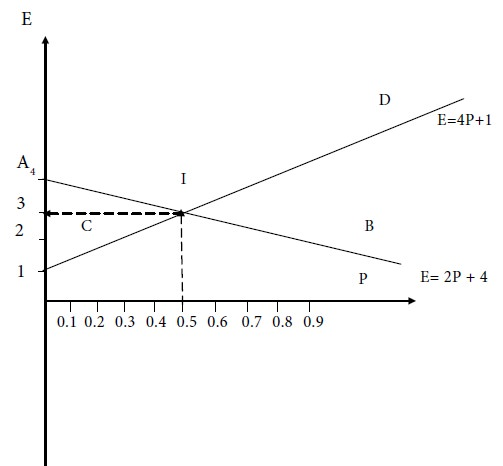Home | ARTS | Operations Management | Graphical solution - Graphical Solution Of A 2X2 Game With No Saddle Point

# Graphical solution - Graphical Solution Of A 2X2 Game With No Saddle Point

Posted On :  25.06.2018 12:16 am

Take probability and expected value along two rectangular axes in a graph sheet.

Graphical solution

## Procedure

Take probability and expected value along two rectangular axes in a graph sheet. Draw two straight lines given by the two equations (1) and (2). Determine the point of intersection of the two straight lines in the graph. This will give the common solution of the two equations (1) and (2). Thus we would obtain the value of the game.

Represent the two equations by the two straight lines AB and CD on the graph sheet. Take the point of intersection of AB and CD as T. For this point, we have p = 0.5 and E = 3. Therefore, the value V of the game is 3.Tags : Operations Management - Game Theory, Goal Programming & Queuing Theory
Last 30 days 98 views